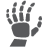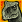cancel
Showing results for
Show  only  | Search instead for
Did you mean:
SOLVED

714 ViewsContributor I

seen to instruction  DIV is 16bit divide by 8bit division instruction. but result is store to 8bit ACCA only.

how can i using this instruction when real 16/8?

Labels (1)
• ### General

1 Solution
570 ViewsNXP Employee

Hi Ki Won Lee,

int x;

char y;

char z;

void main(void) {

...

x= 300;

y = 25;

__asm {

ldhx x;

lda y;

div

}

...

}

6 Replies
570 ViewsContributor III

Hi. the instruction works like this: H:A / X. Must load the 16-bit value (dividend) in H:A as a single record and the divisor in X.

You can use the following:

Load H:X with LDHX instruction and pass the value of X to A. Then load X with the divider 8 bits.

Example:

LDHX #4532

TXA

LDX  #34

DIV

The cocient in ACCA the remainder in H. thus, leading to 0 in A is a decimal add to divide again

Ej2. another way:

LDA  #45

PSHA

PULH

LDA  #32

LDX  #34

DIV

EDiting: I saw a response HX / A. It's wrong. It's not how it works.

570 ViewsContributor I

thanks, yong li,Edward

just catching usage of 8bit DIV instruction.

help from Edward's explanation.

571 ViewsNXP Employee

Hi Ki Won Lee,

int x;

char y;

char z;

void main(void) {

...

x= 300;

y = 25;

__asm {

ldhx x;

lda y;

div

}

...

}

570 ViewsContributor I

thanks Yong li  interest my question.

i would give you ask one more time.

in your example code set  x = 300 and y = 25  maybe get good result 12 in acca . what about y = 1?

acca result is 5?.....  i don't understand this instruction..

570 ViewsSenior Contributor IV

It is very similar to how kids divide 2-digits numbers by 1-digit number on paper. They divide first digit, and then (one only) digit remainder * 10 + next digit. Difference is that we have now bytes instead of decimal digits, rules of math are the same.

unsigned short num= 300;

unsigned char  denom = 25;

unsigned short quotient;

unsigned char remainder;

//DIV instruction   H:A / X = A, rem H

__asm {

ldx denom

clrh

lda num:0; // first digit

div

sta quotient:0

lda num:1 // next digit

div

sta quotient:1

pshh

pula

sta  remainder

}

570 ViewsContributor I

thanks Edward.

then when i using index register high  H ?